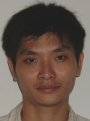Electron. J. Diff. Eqns., Vol. 2005(2005), No. 91, pp. 1-14.

### Oscillation of high order linear functional differential equation with impulses Haihua Liang, Weizhen Feng

Abstract:
We study the solutions to high-order linear functional differential equations with impulses. We improve previous results in the oscillation theory for ordinary differential equations and obtain new criteria on the oscillation of solutions.

Submitted June 28, 2005. Published August 21, 2005.
Math Subject Classifications: 34A37, 34K06, 34K11, 34K25.
Key Words: High order; impulses; differential equation; solution; oscillation.

Show me the PDF file (232K), TEX file, and other files for this article.Haihua Liang School of Mathematical Sciences South China Normal University Guangzhou 510631, China email: haiihuaa@tom.com Weizhen Feng School of Mathematical Sciences South China Normal University Guangzhou 510631, China